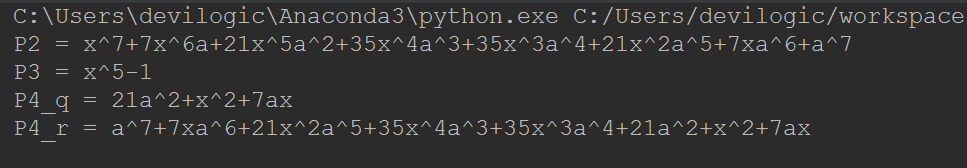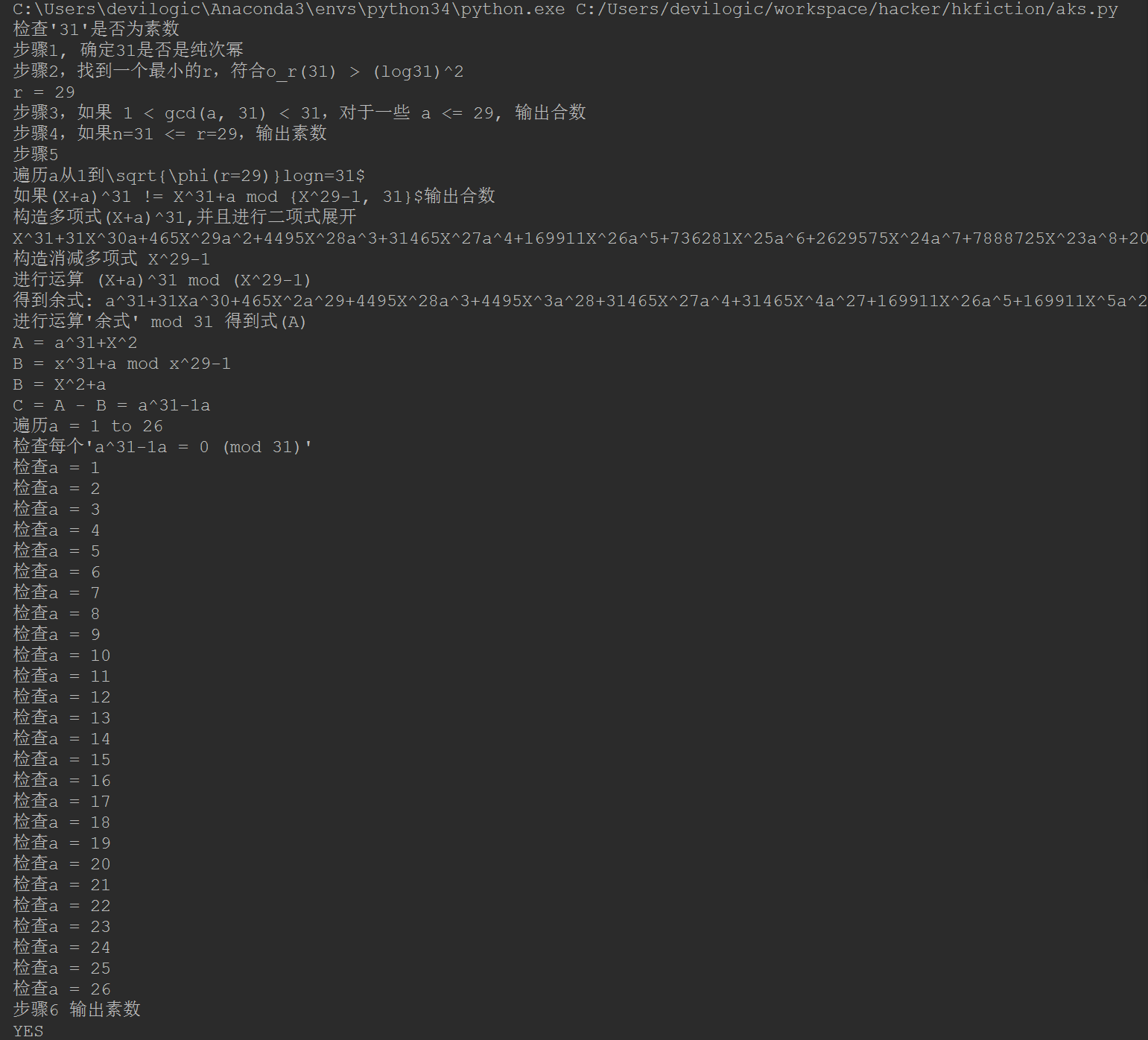@devilogic 2017-10-23T00:35:08.000000Z 字数 18952 阅读 3260

# 玩命的销售日志 2017.10.23

日志 devilogic## 1.介绍

$m \le \lfloor \sqrt{n} \rfloor$。为什么$\lfloor \sqrt{n} \rfloor$是它的界限。一个很简单

$p > \lfloor \sqrt{n} \rfloor$。那么$pq > n$。所以与条件矛盾。

## 2.核心思路

$n$是素数。

$\Omega(n)$表示对于任意一个$c \ge c_0$，则$c_0n \le f(n)$

## 3.标记与预读

$Z_n$表明模$n$的环。$F_p$是模$p$的有限域，其中$p$素数

$n$的操作，保证结果在环$Z_n$中。

$\mathscr N$$\mathscr Z$分别表明自然数集与整数集。给定$r \in \mathscr N$$a \in \mathscr Z$，其中$(a,r)=1$，其中$k$是满足元素$a$$r$群的最小的阶，$a^k \equiv 1 \pmod r$。它使用$o_r(a)$来表示。对于$r \in \mathscr N$$\phi(r)$欧拉函数表示满足所有小于$r$的素数的个数。其中对于每一个$a$$(a,n)=1$，则$o_r(a) \mid \phi(r)$

## 4.算法与证明

1. 如果对于每一个$a \in \mathscr N$$b > 1$，则$n=a^b$，输出合数
2. 找到一个最小的整数$r$，使得$o_r(n)>log^2n$
3. 如果对于一些$a \le r$，则$1 < (a,n) < n$，输出合数
4. 如果$n \le r$，输出素数
5. 循环测试每一个$a=1$$\lfloor \sqrt{\phi(r)}logn \rfloor$，如果$(X+a)^n \not \equiv X^n + a \pmod {X^r - 1, n}$输出合数
6. 输出素数

(保证第二个不等式的所有的$n \ge 2$)，根据引理 3.1，数$B$的第一个最小公倍数至少是$2^B$。因此，$r \le B$

$0 \le a \le l$对于$X+a$在等式$\eqref{e5}$与等式$\eqref{e4}$$\frac{n}{p}$$p$内省的，还是比较清晰的。

$f(X) \in P$，那么

#!/usr/bin/python# coding:utf-8#import numpy as npimport mathimport ysymfrom ysym.ypolynomial import *import gmpy2def is_prime_by_AKS(n):    """    使用AKS算法确定n是否是一个素数    True:n是素数    False:n是合数    """    def __is_integer__(n):        """        判断一个数是否是整数        """        i = int(n)        f = n - i        return not f    def __phi__(n):        """        欧拉函数，测试小于n并与n互素的个数        """        res = n        a = n        for i in range(2, a+1):            if a % i == 0:                res = res // i * (i - 1)                while a % i == 0:                    a //= i        if a > 1:            res = res // a * (a - 1)        return res    def __gcd__(a, b):        """        计算a b的最大公约数        """        if b == 0:            return a        return __gcd__(b, a % b)    print("步骤1, 确定%d是否是纯次幂" % n)    for b in range(2, math.floor(math.log2(n))+1):        a = n**(1/b)        if __is_integer__(a):            return False    print("步骤2，找到一个最小的r，符合o_r(%d) > (log%d)^2" % (n, n))    maxk = math.floor(math.log2(n)**2)    maxr = max(3, math.ceil(math.log2(n)**5))    nextR = True    r = 0    for r in range(2, maxr):        if nextR == False:            break        nextR = False        for k in range(1, maxk+1):            if nextR == True:                break            nextR = (gmpy2.mpz(n**k % r) == 0) or (gmpy2.mpz(n**k % r) == 1)    r = r - 1 # 循环多增加了一层    print("r = %d" % r)    print("步骤3，如果 1 < gcd(a, %d) < %d，对于一些 a <= %d, 输出合数" % (n, n, r))    for a in range(r, 1, -1):        g = __gcd__(a, n)        if g > 1 and g < n:            return False    print("步骤4，如果n=%d <= r=%d，输出素数" % (n, r))    if n <= r:        return True    print("步骤5")    print("遍历a从1到\sqrt{\phi(r=%d)}logn=%d" % (r, n))    print("如果(X+a)^%d != X^%d+a mod {X^%d-1, %d}\$输出合数" % (n, n, r, n))    # 构造P = (X+a)^n mod (X^r-1)    print("构造多项式(X+a)^%d,并且进行二项式展开" % n)    X = multi_ysymbols('X')    a = multi_ysymbols('a')    X_a_n_expand = binomial_expand(ypolynomial1(X, a), n)    print(X_a_n_expand)    X.pow(r)    reduce_poly = ypolynomial1(X, ysymbol(value=-1.0))    print("构造消减多项式 %s" % reduce_poly)    print("进行运算 (X+a)^%d mod (X^%d-1)" % (n, r))    r_equ = ypolynomial_mod(X_a_n_expand, reduce_poly)    print("得到余式: %s" % r_equ)    print("进行运算'余式' mod %d 得到式(A)" % n)    A = ypolynomial_reduce(r_equ, n)    print("A = %s" % A)    print("B = x^%d+a mod x^%d-1" % (n, r))    B = ypolynomial1(multi_ysymbols('X', power=31), a)    B = ypolynomial_mod(B, reduce_poly)    print("B = %s" % B)    C = ypolynomial_sub(A, B)    print("C = A - B = %s" % C)    maxa = math.floor(math.sqrt(__phi__(r)) * math.log2(n))    print("遍历a = 1 to %d" % maxa)    print("检查每个'%s = 0 (mod %d)'" % (C, n))    for a in range(1, maxa+1):        print("检查a = %d" % a)        C.set_variables_value(a=a)        v = C.eval()        if v % n != 0:            return False    print("步骤6 输出素数")    return Trueif __name__ == "__main__":    n = 31    print("检查'%d'是否为素数" % n)    result = is_prime_by_AKS(n)    if result is True:        print("YES")    else:        print("NO")else:    pass

## 5.时间复杂性分析与优化

Artin猜想：给定任意数非完全平方的$n\in \mathscr N$，那么素数的数量$q \le m$对于$o_q(n) = q-1$是逐渐趋向于$A(n)\cdot \frac{m}{lnm}$，这里$A(n)$Artin常数，$A(n) \gt 0.35$

Sophie-Germain素数分布猜想：对于素数个数$q$小于等于$m$，那么$2q+1$仍然是一个素数并且渐进于$\frac{2C_2m}{ln^2m}$，这里$C_2$是孪生素数常量（估计接近$0.66$）。具有这种属性的素数被称为Sophie-Germain素数。

Artin猜想-当$r=O(log^2n)$时对于$m = O\sim(log^2n)$有效。在对Artin猜想的证明已经取得了一些进展[GM84，GMM85，HB86]，这个猜想在一定条件的广义黎曼假设下成立是被承认的。

## 参考资料

[AB03] M. Agrawal and S. Biswas. Primality and identity testing via Chinese remaindering. Jl. of the ACM, 50:429–443, 2003.

[AH92] L. M. Adleman and M.-D. Huang. Primality testing and two dimensional Abelian varieties over finite fields. Lecture Notes in Mathematics, 1512, 1992.

[AKS03] Manindra Agrawal, Neeraj Kayal, and Nitin Saxena. PRIMES is in P. Preprint
(http://www.cse.iitk.ac.in/news/primality v3.ps), February 2003.

[Apo97] T. M. Apostol. Introduction to Analytic Number Theory. Springer-Verlag, 1997.

[APR83] L. M. Adleman, C. Pomerance, and R. S. Rumely. On distinguishing prime numbers from
composite numbers. Ann. Math., 117:173–206, 1983.

[Atk86] A. O. L. Atkin. Lecture notes of a conference, boulder (colorado). Manuscript, August 1986.

[BH96] R. C. Baker and G. Harman. The Brun-Titchmarsh Theorem on average. In Proceedings of a conference in Honor of Heini Halberstam, Volume 1, pages 39–103, 1996.

[BP01] Rajat Bhattacharjee and Prashant Pandey. Primality testing. Technical report, IIT Kanpur,
2001. Available at http://www.cse.iitk.ac.in/research/btp2001/primality.html.

[Car10] R. D. Carmichael. Note on a number theory function. Bull. Amer. Math. Soc., 16:232–238,
1910.

[Fou85] E. Fouvry. Theoreme de Brun-Titchmarsh; application au theoreme de Fermat. Invent. Math.,
79:383–407, 1985.

[GK86] S. Goldwasser and J Kilian. Almost all primes can be quickly certified. In Proceedings of
Annual ACM Symposium on the Theory of Computing, pages 316–329, 1986.

[GM84] R. Gupta and M. Ram Murty. A remark on Artin’s conjecture. Inventiones Math., 78:127–130,
1984.

[GMM85] R. Gupta, V. Kumar Murty, and M. Ram Murty. The Euclidian algorithm for S integers. In
CMS Conference Proceedings, pages 189–202, 1985.

[Gol69] M. Goldfeld. On the number of primes p for which p+a has a large prime factor. Mathematika,
16:23–27, 1969.

[HB86] D. R. Heath-Brown. Artin’s conjecture for primitive roots. Quart. J. Math. Oxford, (2) 37:27–
38, 1986.

[KS02] Neeraj Kayal and Nitin Saxena. Towards a deterministic polynomialtime test. Technical report, IIT Kanpur, 2002. Available at
http://www.cse.iitk.ac.in/research/btp2002/primality.html.

[KSS02] Adam Kalai, Amit Sahai, and Madhu Sudan. Notes on primality test and analysis of AKS.
Private communication, August 2002.

[Lee90] J. V. Leeuwen, editor. Handbook of Theoretical Computer Science, Volume A. Elsevier, 1990.

[Len02] H. W. Lenstra, Jr. Primality testing with cyclotomic rings. Unpublished
(http://cr.yp.to/papers.html#aks has an exposition of Lenstra’s argument), August 2002.

[LN86] R. Lidl and H. Niederreiter. Introduction to finite fields and their applications. Cambridge
University Press, 1986.

[LP03a] H. W. Lenstra, Jr. and Carl Pomerance. Primality testing with gaussian periods. Private
communication, March 2003.

[LP03b] H. W. Lenstra, Jr. and Carl Pomerance. Remarks on Agrawal’s conjecture. Unpublished
(http://www.aimath.org/WWN/primesinp/articles/html/50a/), March 2003.

[Mac02] Martin Macaj. Some remarks and questions about the AKS algorithm and related conjecture.
Unpublished (http://thales.doa.fmph.uniba.sk/macaj/aksremarks.pdf), December 2002.

[Mil76] G. L. Miller. Riemann’s hypothesis and tests for primality. J. Comput. Sys. Sci., 13:300–317,
1976.

[Nai82] M. Nair. On Chebyshev-type inequalities for primes. Amer. Math. Monthly, 89:126–129, 1982.

[Pra75] V. Pratt. Every prime has a succinct certificate. SIAM Journal on Computing, 4:214–220,
1975.

[Rab80] M. O. Rabin. Probabilistic algorithm for testing primality. J. Number Theory, 12:128–138,
1980.

[SS77] R. Solovay and V. Strassen. A fast Monte-Carlo test for primality. SIAM Journal on Computing, 6:84–86, 1977.

[vzGG99] Joachim von zur Gathen and J¨urgen Gerhard. Modern Computer Algebra. Cambridge University Press, 1999• 私有
• 公开
• 删除